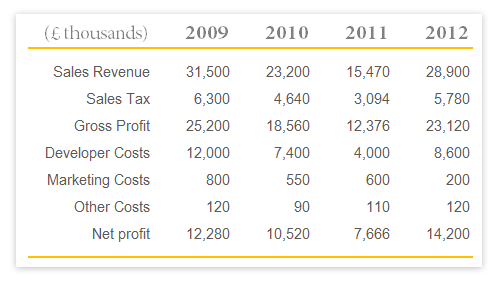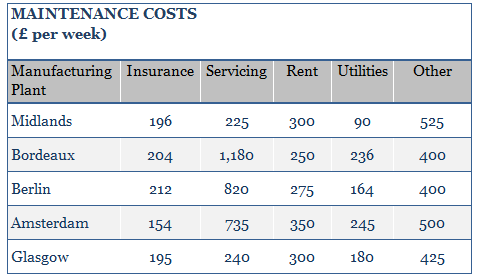# Category Archives: Ratios

## Classic Question 1: If sales revenue in 2011 was split between online and offline sales in the ratio 7:2, what was the revenue from offline sales in 2011 (to the nearest £100)?Step 1: Analyse the problem

From the table we see that the sales revenue in 2011 was 15,470 (£ thousands).

We are told that online:offline sales are in the ratio of 7:2. And it is safe to assume there are no other categories (i.e. we assuming all sales are either online or offline, nothing else). So the offline portion of these sales will be 2 out of a total of 9 (7 + 2).

Step 2: Apply the ratio

Offline sales will be 2 ÷ (7 + 2) x 15,470 = 3,437.78

Ans = £3,437,800

Note that we divided by 9 because we want the fraction of offline sales relative to all sales revenue.

## Classic Question 2: Which manufacturing plant had the lowest ratio of Servicing to Utilities, and what was this ratio?Step 1: Quickly scan the data to see if any of the options are obviously not correct, thus saving you time by not having to calculate them. Personally, I can’t see an obvious contender to leave out, so I will calculate all five.

Step 2: For all five manufacturing plants divide Service by Utilities:

Midlands:  225 ÷ 90 = 2.5
Bordeaux:  1,180 ÷ 236 = 5.0
Berlin  820 ÷ 164 = 5.0
Amsterdam  735 ÷ 245 = 3.0
Glasgow  240 ÷ 180 = 1.3333

Warning: make sure you get the numbers the right way round when you are dividing one by the other.

Step 3: Convert to a fractions.

We can see that part of the answer is Glasgow because it has the lowest number, now we just need to convert 1.333(recuring) to a fraction. Whilst some scientific calculators will turn 1.333 recurring into a fraction, there are certain fractions you will be expected to know. This is one of them and you should be able to see that this is 4/3.

Ans: Glasgow 4:3

Other common ratios you would be expected to recognise are:

0.1 = 1/10
0.2  = 1/5
0.25 = 1/4
0.33 recurring = 1/3
0.5 = 1/2